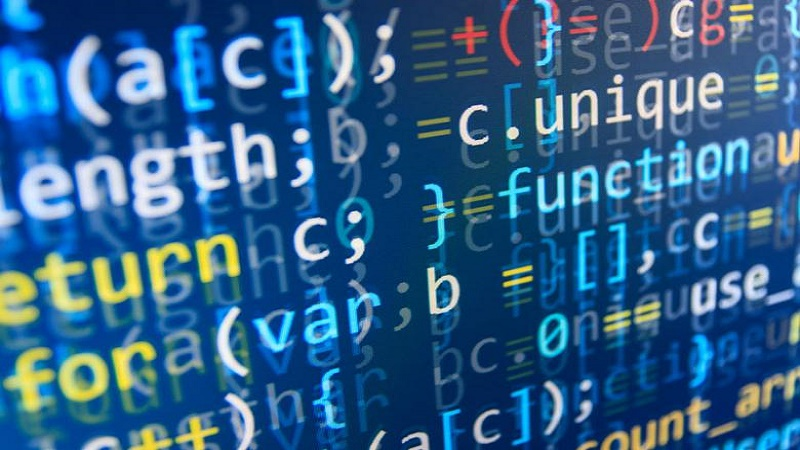Problem Solving and Python Programming - GE8151

Online Study Material, Lecturing Notes, Assignment, Reference, Wiki and important questions and answersUNIT I Algorithmic Problem Solving

=> Problem Solving Techniques
=> Algorithm
=> Building blocks of algorithms (statements, state, control flow, functions)
=> Notation
=> Flow chart
=> Pseudo code
=> programming language
=> Categories of programming languages
=> Algorithmic problem solving
=> Simple Strategies For Developing Algorithms
=> Examples algorithms: pseudo code, flow chart, programming language
=> Basic python programs
=> Python Algorithmic Problem Solving: short important questions and answers
=> Python Algorithmic Problem Solving: brief important questions and answers

UNIT II Data Expressions Statements

=> Introduction to Python
=> Python interpreter
=> Modes of Python Interpreter
=> Values and Data Types
=> Variables - Python
=> Keywords - Python
=> Identifiers - Python
=> Statements and Expressions - Python
=> Input and Output - Python
=> Docstring - Python
=> Lines and Indentation - Python
=> Quotation in Python
=> Tuple Assignment - Python
=> Operators and Types of Operators - Python
=> Operator Precedence - Python
=> Functions - Python
=> Types of function
=> Function definition(Sub program) - Python
=> Flow of Execution - Python
=> Function Prototypes
=> Parameters and Arguments - Python
=> Modules - Python
=> Python Programs - Data, Expressions, Statements
=> Python Data, Expressions, Statements: short important questions and answers
=> Python Data, Expressions, Statements: brief important questions and answers

UNIT III Control Flow Functions

=> Boolean Values
=> Conditionals - Python
=> Iteration/Control Statements - Python
=> Fruitful Function - Python
=> Strings - Python
=> List as array - Python
=> Example Python Programs: Control Flow, Functions
=> Python Control Flow, Functions: short important questions and answers
=> Python Control Flow, Functions: brief important questions and answers

UNIT IV Lists Tuples Dictionaries

=> Lists - Python
=> Tuple - Python
=> Dictionaries - Python
=> Difference between List, Tuples and dictionary
=> Advanced list processing - Python
=> Python Programs on matrix
=> Python Programs on Lists, Tuples, Dictionaries
=> Python Lists, Tuples, Dictionaries: short important questions and answers
=> Python Lists, Tuples, Dictionaries: brief important questions and answers

UNIT I Algorithmic Problem Solving

=> Programming Algorithm
=> Flowcharts
=> Guidelines For Drawing a Flowchart
=> Pseudocode
=> Example Programming Algorithm, Pseudocode, Flowchart

UNIT II Data Expressions Statements

=> What is a program?
=> Python programming language
=> Values and types - Python
=> Expressions and statements - Python
=> Python Tuple
=> Python Strings
=> Python Output Using print() function
=> Modules - Python
=> Python Import
=> Operators and operands
=> Type of operators in Python
=> Functions - Python
=> Flow of execution - Python
=> Parameters and arguments - Python
=> Python Basic Programs

UNIT III Control Flow Functions

=> Conditionals - Python
=> Recursion - Python
=> Iteration - Python
=> Fruitful Functions - Python
=> Strings - Python
=> Example Python Programs on Control Flow, Functions

UNIT IV Lists Tuples Dictionaries

=> Lists - Python
=> Tuples - Python
=> Python dictionary
=> Example Python Programs on Lists, Tuples, Dictionaries

UNIT V Files Modules Packages

=> Files - Python
=> Exception - Python
=> Modules - Python
=> Package - Python
=> Python Files, Modules, Packages: short important questions and answers
=> Example Python Programs on Files, Modules, Packages

GE8151 Problem Solving and Python Programming - Anna University 2017 Regulation Syllabus - Download Pdf
GE8151 Problem Solving and Python Programming - Question Bank - Download Pdf
GE8151 Problem Solving and Python Programming - Question Paper 2017 - Download Pdf

GE8151 Problem Solving and Python Programming - Question Paper Jan 2018
Problem Solving and Python Programming - Start Reading Online
265 - 1st Year Important Questions with Answers
265 - GE8151 Problem Solving and Python Programming - Notes 1.pdf
265 - GE8151 Problem Solving and Python Programming - Notes 2.pdf
265 - GE8151 Problem Solving and Python Programming - Question Bank 2.pdf
265 - GE8151 Problem Solving and Python Programming - Question Bank.pdf
265 - GE8151 Problem Solving and Python Programming - 2 marks with Answers.pdf
265 - GE8151 Problem Solving and Python Programming - Question Bank 3.pdf

265 - GE8151 Problem Solving and Python Programming - Dec Jan 2019 Question Papers.pdf
265 - GE8151 Problem Solving and Python Programming - Dec 2017 Jan 2018 Question Paper.pdf